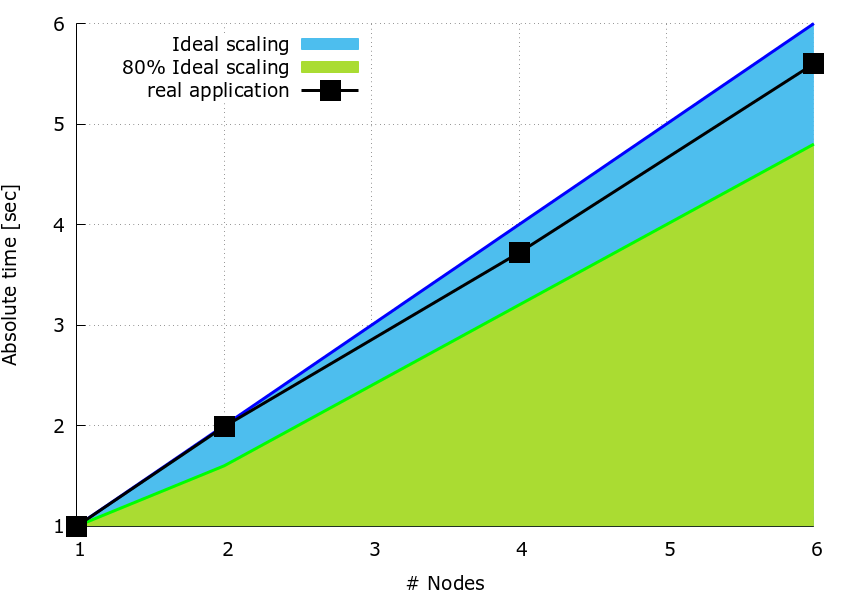# Tuning BLAS routines invocation (gemm)

Pattern addressed: Low computational performance calling BLAS routines (gemm)

In many scientific applications, parallel matrix-matrix multiplications represent the computational bottleneck. Consider the following matrix-matrix multiplication: (more...)

In many scientific applications, parallel matrix-matrix multiplications represent the computational bottleneck. Consider the following matrix-matrix multiplication:

$\bf {C}= \bf {A} \times \bf {B}$

where, $$\bf {A}$$ is a matrix with $$N_m$$ rows and $$N_k$$ columns (i.e. $$N_m \times N_k$$), $$\bf {B}$$ is a $$N_k \times N_n$$ matrix so that $$\bf {C}$$ is a $$N_m \times N_n$$ matrix.

In the case each process has access to $$\bf {A}$$ and $$\bf {B}$$, a strategy to parallelise the work is to divide $$\bf {A}$$ and/or $$\bf {B}$$ into blocks to partition the computation over all processes, and use BLAS routines, e.g. dgemm, on the individual block multiplications.

It can be observed that different partitioning of the matrices $$\bf {A}$$ and $$\bf {B}$$ can result in different computational performance due to different values of IPC (instructions per cycle). For example, splitting $$\bf {B}$$ over the columns and leaving $$\bf {A}$$ unchanged can result in poor computational scaling (see the related pattern addressed).

When such poor parallel scaling occurs, it is best practice to write a simple code in order to test alternative partitioning of the matrix multiplication. In the case described above, where $$\bf {B}$$ is split over the columns, and $$\bf {A}$$ is not split, the size of the matrix blocks is determined by the number of processes. However, if we split $$\bf {A}$$ over the rows, whilst still splitting $$\bf {B}$$ over the columns, we have a choice of the size of the matrix blocks for $$\bf {A}$$ (i.e. $$N_v=N_m/n_{parts_A}$$) and of $$\bf {B}$$ (i.e. $$N_w=N_n/n_{parts_B}$$), where $$n_{parts_A}$$ and $$n_{parts_B}$$ are two integers and their product would typically equal the number of processes.

The calculation of a block of matrix $$\bf {C}$$, defined as $$\bf {C_{v,w}}$$ with size $$N_v \times N_w$$, can be expressed as:

$\bf {C_{v,w}} = \bf {A_v} \times \bf {B_w}$

By experimenting with blocks of $$\bf {C_{v,w}}$$ having different values of $$n_{parts_A}$$ and $$n_{parts_B}$$, namely different values for columns ($$N_w$$) and rows ($$N_v$$), we can find out optimal pairs.

The pseudo-code implementation of the aforementioned algorithm can be:

  given:
N_m, N_n, N_k, n_parts_B

execute:
get_my_process_id(proc_id)
get_number_of_processes(n_proc)

DEFINE first_b, last_b, len_n_part AS INTEGER
DEFINE first_a, last_a, n_parts_A, len_m_part AS INTEGER
DEFINE alpha, beta AS DOUBLE

first_b = float(N_n) * proc_id / n_parts_B
last_b = (float(N_n) * (proc_id + 1) / n_parts_B) - 1

n_parts_A = n_proc / n_parts_B

first_a = float(N_m) *  proc_id / n_parts_A
last_a = (float(N_m) * (proc_id + 1) / n_parts_A) - 1

len_n_part = last_b - first_b + 1;
len_m_part = last_a - first_a + 1;

SET alpha TO 1.0
SET beta TO 0.0
call dgemm('N', 'N', len_m_part, len_n_part, N_k, alpha, A[:,first_a:last_a], len_m_part, B[:,first_b:last_b], N_k, beta ,C, len_m_part)


Speedup tests

The speedup plot below is from a simple timing code written to test the strategy of dividing both matrices, $$\bf{B}$$ and $$\bf{A}$$, into blocks, and splitting the individual multiplications over the processes using the sequential dgemm subroutine from MKL-2017. The code doesn’t actually implement the parallel matrix-matrix multiplication, it simply replicates the same computation for one node. The proposed best-practice is meant to show how a simple code can be used to compare the timing for different splitting on $$nNodes$$ nodes relative to one node and to identify the best partition.

The three matrices have size $$N_n \times N_n$$ where $$N_n = 3600$$. On one node, the number of columns for $$\bf{B_w}$$ is $$N_w=N_n/ (2 \times nNodes)$$ while the number of rows of $$\bf{A_v}$$ is $$N_v=N_m/24$$ for all cases.

The speedup plot in Figure 1, is obtained by using the sequential dgemm with parameters alpha and beta are set to $$1.0$$ and $$0.0$$, respectively.

The code was run on a fully populated compute node of MN4 i.e. using 48 processes, the computation was repeated $$100 \times$$ to give measurable timings.Figure 1: speedup plot.

In contrast to the pattern, it can be observed how the speedup of each run is always higher than $$80 \%$$ with respect to the ideal.

Recommended in program(s): Low computational performance calling BLAS routines ·

Implemented in program(s): Tuning BLAS routines invocation ·